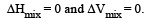Courses

# Test: Solutions 1 - From Past 28 Years Questions

## 17 Questions MCQ Test Chemistry 28 Years Past year papers for NEET/AIPMT Class 12 | Test: Solutions 1 - From Past 28 Years Questions

Description
This mock test of Test: Solutions 1 - From Past 28 Years Questions for Class 12 helps you for every Class 12 entrance exam. This contains 17 Multiple Choice Questions for Class 12 Test: Solutions 1 - From Past 28 Years Questions (mcq) to study with solutions a complete question bank. The solved questions answers in this Test: Solutions 1 - From Past 28 Years Questions quiz give you a good mix of easy questions and tough questions. Class 12 students definitely take this Test: Solutions 1 - From Past 28 Years Questions exercise for a better result in the exam. You can find other Test: Solutions 1 - From Past 28 Years Questions extra questions, long questions & short questions for Class 12 on EduRev as well by searching above.
QUESTION: 1

### During osmosis, flow of water through a semi-permeable membrane  

Solution:

During osmosis, flow of water through a semi-permeable membrane is from solution having lower concentration only.

QUESTION: 2

### A solution containing 10 g per dm 3 of urea (molecular mass = 60 g mol–1) is isotonic with a 5% solution of a  non-volatile solute. The molecular mass of this nonvolatile solute is 

Solution:

Osmotic pressure of urea from the formula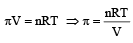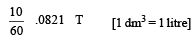5% solution means
100 ml     5gm
1000 ml    50 g/L
Osmotic pressure of solution having nonvolatile solute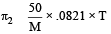For isotonic solution,   π12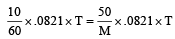M = 300 gm mol–1

QUESTION: 3

### 1.00 g of a non-electrolyte solute (molar mass 250 g mol–1) was dissolved in 51.2 g of benzene. If the freezing point depression constant, Kf of benzene is 5.12 K kg mol–1, the freezing point of benzene will be lowered by 

Solution: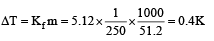QUESTION: 4

0.5 molal aqueous solution of a weak acid (HX) is 20% ionised. If Kf for water is 1.86 K kg mol– 1,the lowering in freezing point of the solution is

Solution:

As ΔTf = iKfm

For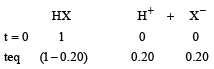Total no. of moles = 1 – 0.20 + 0.20 + 0.20 = 1 + 0.20 = 1.2
∴ ΔTf = 1.2 × 1.86 × 0.5 = 1.1160 ≈ 1.12 K

QUESTION: 5

A 0. 00 20 m aqueous solution of an ionic compound Co(NH3)5(NO2)Cl freezes at – 0.00732 °C. Number of moles of ions which 1 mol of ionic compound produces on being dissolved in water will be (Kf = – 1.86°C/m)

Solution:

ΔTf = 0 – (0.00732°) = 0.00732
ΔTf = i × Kf × m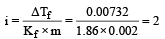QUESTION: 6

An aqueous solution is 1.00 molal in KI. Which change will cause the vapour pressure of the solution to increase? 

Solution:

When the aqueous solution of one molal KI is diluted with water, concentration decreases, therefore the vapour pressure of the resulting solution increases.

QUESTION: 7

A solution of sucrose (molar mass = 342 g mol–1) has been prepared by dissolving 68.5 g of sucrose in 1000 g of water. The freezing point of the solution obtained will be ( f for water = 1.86 K kg mol–1). 

Solution: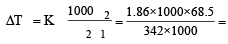0.372
T = – 0.372°C

QUESTION: 8

2 5. 3 g of sodium carbonate, Na2 CO3 is dissolved in enough water to make 250 mL of solution. If sodium carbonate dissociates completely, molar concentration of sodium ions, Na+ and carbonate ions, CO32– are respectively (Molar mass of Na2CO3 = 106 g mol–1) 

Solution:

Concentration of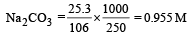[Na+] = 2 × 0.955 = 1.91 M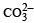= 0.955 M

QUESTION: 9

The freezing point depression constant for water is – 1.86ºC m–1. If 5.00 g Na2SO4 is dissolved in 45.0 g H2O, the freezing point is changed by – 3.82ºC. Calculate the van’t Hoff factor for Na2SO4. 

Solution:

Given Kf = – 1.86º cm–1, mass of solute = 5.00 g, mass of solvent = 45.0 g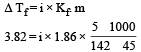∴ i = 2.63 (Molecular mass of Na2SO4 = 142)

QUESTION: 10

The van’t Hoff factor i for a compound which undergoes dissociation in one solvent and association in other solvent is respectively : 

Solution:

If compound dissociates in solvent i > 1 and on association i < 1.

QUESTION: 11

Mole fraction of the solute in a 1.00 molal aqueous solution is : 

Solution:

1 molal solution means 1 mole of solute dissolved in 1000 gm solvent.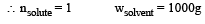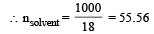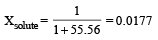QUESTION: 12

A 0.1 molal aqueous solution of a weak acid is 30% ionized. If Kf for water is 1.86°C/m, the freezing point of the solution will be : [2011 M]

Solution:

Given α = 30% i.e., 0.3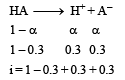i = 1.3
ΔTf = 1.3 × 1.86 × 0.1 = 0.2418
Tf = 0 – 0.2418 = – 0.2418 °C

QUESTION: 13

200 mL of an aqueous solution of a protein contains its 1.26 g. The osmotic pressure of this solution at 300 K is found to be 2.57 × 10–3 bar.The molar mass of protein will be (R = 0.083 L bar mol–1 K–1)            [2011 M]

Solution:

(π) = CRT Osmotic pressure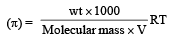= 2.57 10 3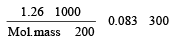Molecular mass = 61038 g

QUESTION: 14

PA and PB are the vapour pressure of pure liquid components, A and B, respectively of an ideal binary solution. If XA represents the mole fraction of component A, the total pressure of the solution will be. 

Solution: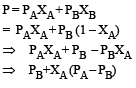QUESTION: 15

Vapour pressure of chloroform (CHCl3) and dichloromethane (CH2Cl2) at 25ºC are 200 mm Hg and 41.5 mm Hg respectively. Vapour pressure of the solution obtained by mixing 25.5 g of CHCl3 and 40 g of CH2Cl2 at the same temperature will be : (Molecular mass of CHCl3 = 119.5 u and molecular mass of CH2Cl2 = 85 u).             [2012 M]

Solution:
QUESTION: 16

How many grams of concentrated nitric acid solution should be used to prepare 250 mL of 2.0M HNO3 ? The concentrated acid is 70% HNO3             [NEET 2013]

Solution:

Molarity (M)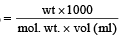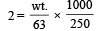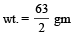wt. of 70% acid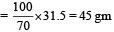QUESTION: 17

Which condition is not satisfied by an ideal solution? [NEET Kar. 2013]

Solution:

An ideal solution is that solution in which each component obeys Raoult’s law under all conditions of temperatures and concentrations. For an ideal solution.